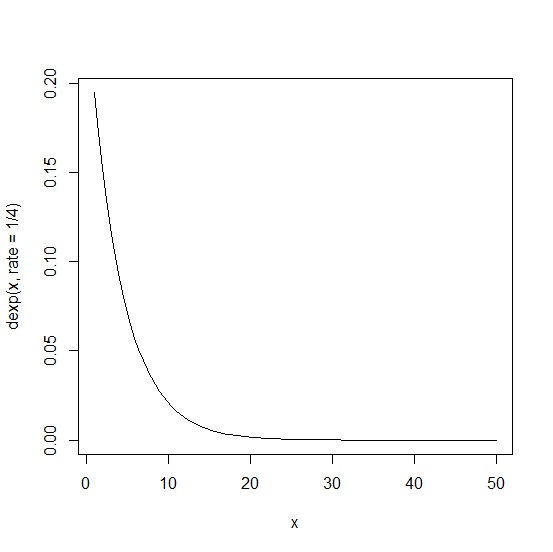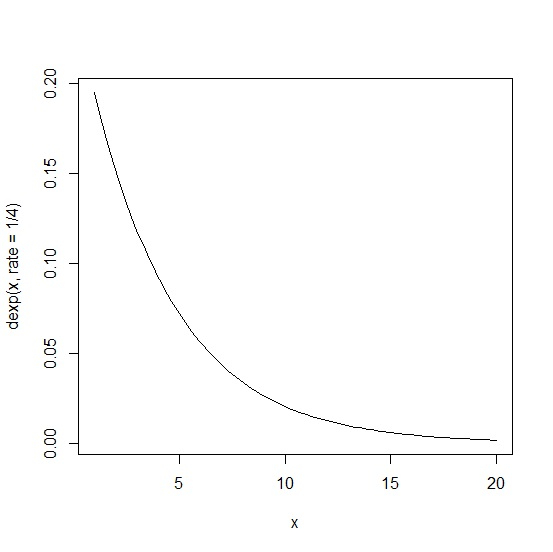# How to create an exponential distribution plot in R?

To create an exponential distribution plot, we can use curve function.

For example, if we want to create a exponential distribution plot for 100 values with rate parameter equal to ½ then we can use the command given below:

curve(dexp(x,rate=1/2),xlim=c(1,50))

Check out the below examples to understand how it works.

## Example 1

To create an exponential distribution plot, use the command given below −

curve(dexp(x,rate=1/4),xlim=c(1,50))


## Output

If you execute all the above given snippets as a single program, it generates the following output: −## Example 2

To create an exponential distribution plot, use the command given below −

curve(dexp(x,rate=1/4),xlim=c(1,20))

## Output

If you execute all the above given snippets as a single program, it generates the following output: −